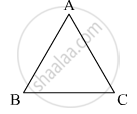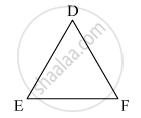# In Two Triangles Abc and Def, It is Given that ∠A = ∠D, ∠B = ∠E and ∠C =∠F. Are the Two Triangles Necessarily Congruent? - Mathematics

In two triangles ABC and DEF, it is given that ∠A = ∠D, ∠B = ∠E and ∠C =∠F. Are the two triangles necessarily congruent?

#### Solution

It is given that ∠A = ∠D , ∠B = ∠E , ∠C = ∠FFor necessarily triangle to be congruent, sides should also be equal.

Concept: Criteria for Congruence of Triangles
Is there an error in this question or solution?

#### APPEARS IN

RD Sharma Mathematics for Class 9
Chapter 12 Congruent Triangles
Exercise 12.7 | Q 2 | Page 84
Share